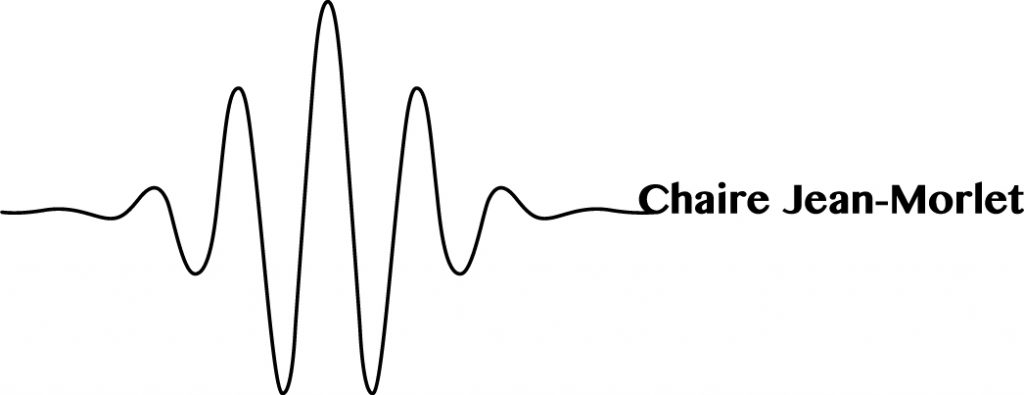## RESEARCH IN RESIDENCE

#### Discrete Fracture Networks 5-9 December 2022#### Participants

Martin Gander (Université de Genève)​
Julian Hennicker (Université de Genève)
Roland Masson (Université Côte d’Azur)
Tommaso Vanzan (École Polytechnique fédérale de Lausanne)

Discrete fracture networks are models to simulate flow behavior in the underground in the presence of fractures. In the fractures, the flow behavior is very different from the flow in the so-called surrounding matrix. The simulation of one fracture thus requires both a different flow model in the fracture from the flow model in the matrix, and coupling conditions between the thin fracture and the matrix. In addition, the mesh, in general, needs to be extremely fine in the fracture compared to the matrix. While this is possible for the simulation of one (or a few fractures), it becomes prohibitive when many fractures are present. In the limit of a very large number, one could then use homogenization techniques, but we are interested here in the intermediate, hard regime, where the fractures still need to be modeled individually, and there are many of them. A common method to establish such models consists in integrating the fracture equations over the fracture width and using some ad hoc approximations for the coupling conditions [2, 5, 1, 4]. We are interested here in a systematic construction of reduced order elliptic PDE models for such fractured domains. In , we derived coupling conditions between matrix subdomains, when the fracture is represented as a hypersurface embedded in the surrounded rock matrix. These coupling conditions were obtained by analytically eliminating the fracture and encoding the information of the fracture in the coupling conditions via a continuous variant of a Schur complement. We then derived local approximations of these coupling conditions using asymptotic expansions when 1 the fracture width goes to zero. A complete analysis of this approach can be found in . In the present project, we are interested in a different asymptotic regime, namely a fixed small fracture width, but when the mesh size of the numerical approximation is going to zero. The advantage of this second approach is that it will be suitable also for fractures with a more realistic fracture width, and at the same time optimized for the numerical method used. We will follow in our approach the analogy we know from domain decomposition methods, in particular optimized Schwarz methods, where for transport dominated flows, optimized transmission conditions were derived both for the physical parameter represented by the Peclet number becoming large, or the numerical mesh parameter becoming small.